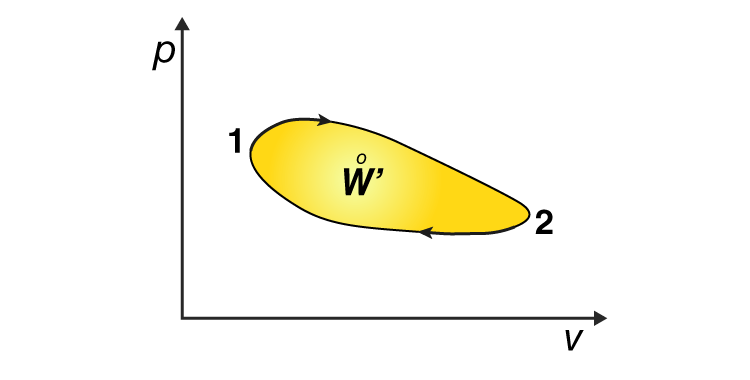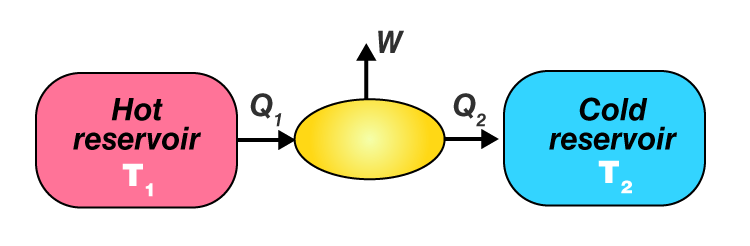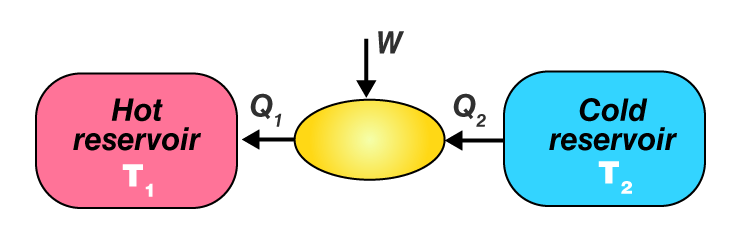# Cyclic Process

## What Is Cyclic Process?

The process in which the initial and final state is the same is known as a cyclic process. It is a sequence of processes that leave the system in the same state in which it started.When a system undergoes a cyclic process, its initial and final internal energies are equal. Hence, the internal energy change in any cyclic process is zero. Applying the first law of thermodynamics to a cyclic process, we get

$$\begin{array}{l}\Delta E=Q+W\end{array}$$

As

$$\begin{array}{l}\Delta E=0\end{array}$$
,

$$\begin{array}{l}W=Q\end{array}$$

Hence, the work done by the system in a cyclic transformation is equal to the heat absorbed by the system. The net work involved in a cyclic process is the area enclosed in a P-V diagram. If the cycle goes clockwise, the system does work. If the cycle goes anticlockwise, then the work is done on the system every cycle. An example of such a system is a refrigerator or air conditioner. The Carnot engine is the best example of a cyclic process.

## What Is Heat Engine?

A heat engine is a device by which a system is made to undergo a cyclic process that results in the conversion of heat to work.

• Heat engines consist of a working substance– the system. For example, steam in a steam engine is the working substance.
• The working substance goes through a cycle of several processes. In some processes, it absorbs heat from a reservoir at a high temperature.
• In some processes, the working substance releases some amount of heat to an external reservoir at a lower temperature.
• The work done by the system in a cycle is transferred to the environment through some arrangements. For example, the working substance may be in a cylinder with a moving piston that transfers mechanical energy to the wheels of the vehicle.

The schematic representation of a heat engine is represented as follows:The cycle is repeated and again to get the useful work done for some purpose.

### What Is Refrigerator?

A refrigerator is a reverse of the heat engine. Here, the working substance extracts some amount of heat from the cold reservoir at some temperature T1. An external work W is done on it and heat Q is released to the hot reservoir at a temperature T2. The schematic representation of a refrigerator is given below.Related Articles

Stay tuned to BYJU’S to learn more physics concepts like the working of refrigerator and heat pumps with the help of interactive videos.

Test your knowledge on Cyclic process